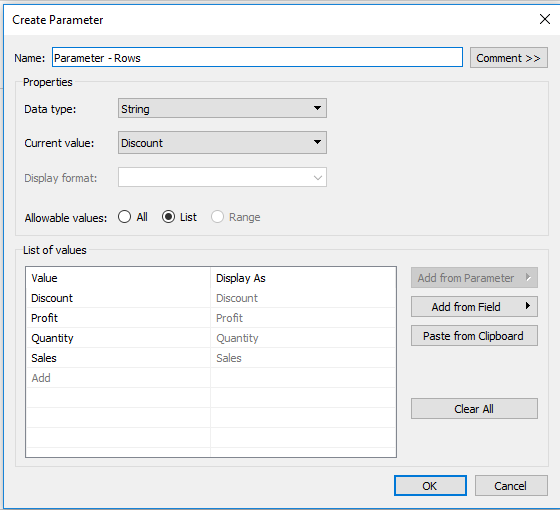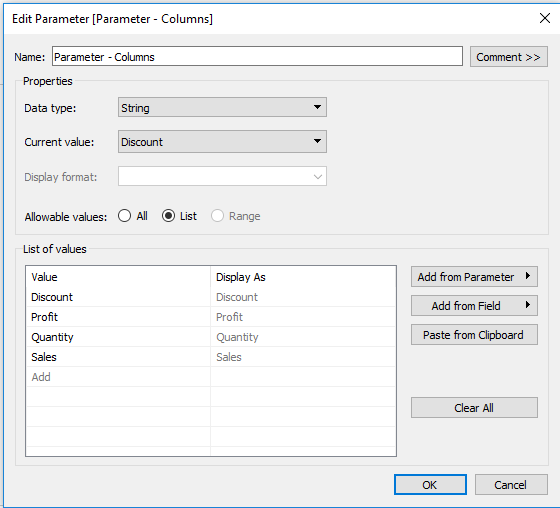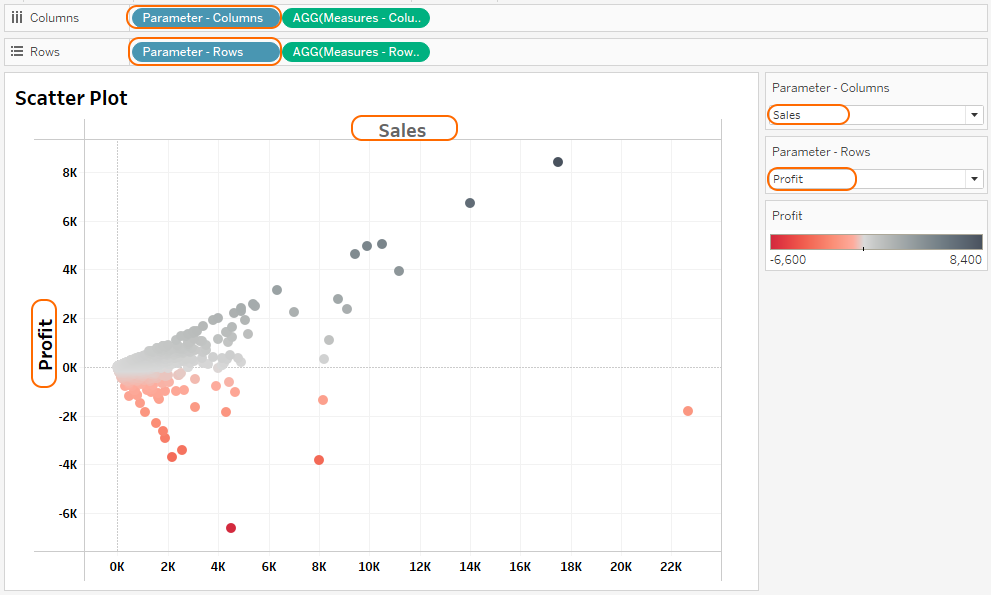Search
• Bernard

# Tableau Tips: How To Create A Dynamic Scatter Plot

Updated: Aug 18Scatter plot is a data viz, that helps users explore the relationship between measures. In Tableau, a scatter plot is built by placing one measure field in the rows shelf and another measure field in the columns shelf.

Note: Setting your measure fields as described above creates a scatter plot by default. However, it’s good to note the level of detail in which the scatter plot is computed as we will describe below.

### Creating a simple scatter plot

Using Orders table of the Superstores data set, a simple scatter plot using the measure field Profit and Sales could be created as follows;

• Drag measure field Profit to the rows shelf.

• Drag measure field Sales to the columns shelf.

Note: This results to a view with a single dot.Why the single dot in our scatter plot? Because we’ve not specified the level of detail for each point to be plotted. This can be done in two ways;

1. Plotting every data point in the data set by opening the analytics menu and uncheck the field ‘Aggregate Measures’.

2. Dragging the dimension of interest to the details shelf. For this case, level of detail will be defined using ‘State’.Creating a scatter plot is simple as demonstrated above. And looking at one of the scatter plot’s above, it does not give us the flexibility of changing the measures applied in the view. (Applicable when dealing with multiple measure fields).(In the above scatter plot, we can only consume the viz as per the present measure fields, to add some dynamics, we’ll require to use a parameter).

### Let’s Parameterize our scatter plot

Create a parameter field named ‘Parameter – Rows’ to help us change fields in the rows shelf.Repeat the same (duplicate the parameter) to create a parameter field named ‘Parameter – Columns’ to help us change fields in the columns shelf.Now parameters are ready, but they can’t be used in our viz because they are not tied to the measure fields. We’ve to create the following two calculated fields to link the parameters with measure fields.### Build now a dynamic scatter plot

• Drag measure field ‘Measures - Rows’ to the rows shelf.

• Drag measure field ‘Measures – Columns’ to the columns shelf.

• Under the analytics menu uncheck the field ‘Aggregate Measures’.

• Show parameter controls.

Note: Any changes made on the parameters is now reflected on the viz.### Pro-tip

Note: The axis title of the scatter plot remains to be ‘Measures – Rows’ and ‘Measures – Columns’ irrespective of the field selected in the parameters.

This can be corrected by adding the parameter fields ‘Parameter – Rows’ and ‘Parameter – Columns’ to the rows and columns shelf respectively, and deleting the initial title by editing the axis.A sample dashboard build on the same methodology can be accessed here.